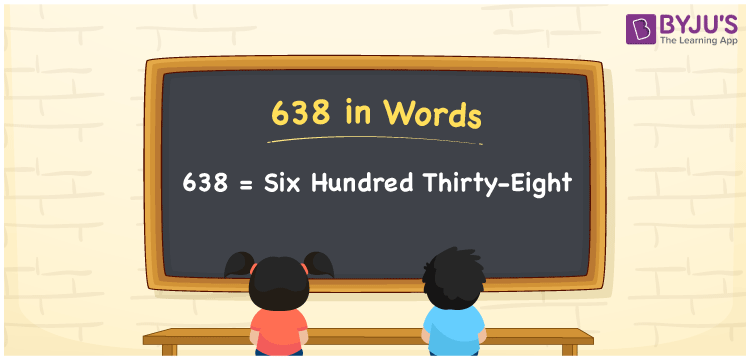# 638 in words

638 in words is written as Six Hundred and Thirty Eight. 638 represents the count or value. The article on Counting Numbers can give you an idea about count or counting. The number 638 is a 3 digit number that is used in expressions related to money, days, distance, length, weight and so on. Let us consider an example for 638. “There are Six Hundred and Thirty Eight people visiting the memorial.”

 638 in words Six Hundred and Thirty Eight Six Hundred and Thirty Eight in Numbers 638

## 638 in English Words## How to Write 638 in Words?

We can convert 638 to words using a place value chart. The number 638 has 3 digits, so let’s make a chart that shows the place value up to 3 digits.

 Hundreds Tens Ones 6 3 8

Thus, we can write the expanded form as:

6 × Hundred + 3 × Ten + 8 × One

= 6 × 100 + 3 × 10 + 8 × 1

= 638

= Six Hundred and Thirty Eight.

638 is the natural number that is succeeded by 637 and preceded by 639.

638 in words – Six Hundred and Thirty Eight.

Is 638 an odd number? – No.

Is 638 an even number? – Yes.

Is 638 a perfect square number? – No.

Is 638 a perfect cube number? – No.

Is 638 a prime number? – No.

Is 638 a composite number? – Yes.

## Solved Example

1. Write the number 638 in expanded form

Solution: 6 × 100 + 3 × 10 + 8 × 1

We can write 638 = 600 + 30 + 8

= 6 × 100 + 3 × 10 + 8 × 1.

## Frequently Asked Questions on 638 in words

Q1

### How to write the number 638 in words?

638 in words is written as Six Hundred and Thirty Eight.
Q2

### Is 638 divisible by 3?

No. 638 is not divisible by 3.
Q3

### Is 638 a perfect square number?

No. 638 is not a perfect square number.